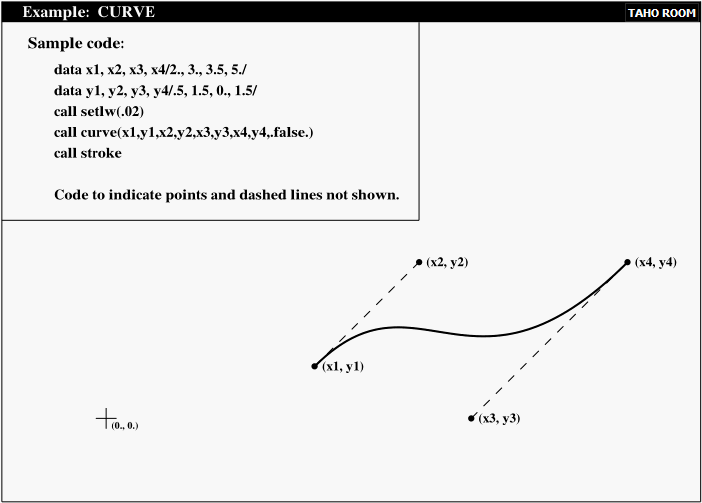### —————————————————————————-

I recommend the PSPLOT package written by Mr Kevin Kohler
(http://www.nova.edu/cwis/oceanography/psplot.html)
The ready-to-use binary form of the library is available from:

http://www.geocities.com/Athens/Olympus/5564/

The PSPLOT library is free to use for non-commercial purposes.

### —————————————————————————-

PSPLOT  http://www.nova.edu/cwis/oceanography/psplot.html
PostScript for Technical Drawings
A free Fortran-callable PostScript Plotting Library
Written by: Kevin E. Kohler

[Introduction]
[Plotting Basics]
[Subroutines]
[Output Example: Black and White]
[Output Example: Color]
[Obtaining PSPLOT]

URPOSE: CURVE draws a curve between two points, given the beginning and ending curve slopes.

SYNTAX: CALL CURVE (X1, Y1, X2, Y2, X3, Y3, X4, Y4, CONTIN)

ARGUMENTS:

X1,Y1 X,Y coordinates of the beginning of the curve.

X2,Y2 X, Y coordinates specifying the beginning curve slope. The initial slope of the curve is given by (Y2-Y1)/(X2-X1).

X3,Y3 X,Y coordinates specifying the ending curve slope. The end slope of the curve is given by (Y4-Y3)/(X4-X3).

X4,Y4 X,Y coordinates of the endpoint of the curve.

CONTIN Logical value. If CONTIN=.false. then the curve drawn starts a newpath; otherwise, the curve is appended to the current path.

Note: The curve produced by CURVE is not automatically stroked.

For example: